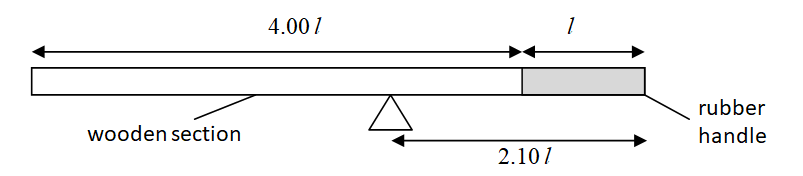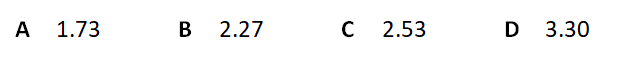# Question Guide – Turning Effect of Forces and Density

Question:
A uniform rod has a wooden section and a solid rubber handle, as shown in the diagram below.
The length of the handle is l and the length of the wooden section is 4.00 l. The rod balances at a distance of 2.10 l from the rubber end.Assume that the density of wood is approximately 1.01 g/cm3.
What is the density of rubber?Solution:

The Centre of Gravity (CG) of a uniform body is at the centre of the body.

Thus, the CG of the wood section is atTherefore, the CG of the wood section from the pivot,The CG of the rubber section is atTherefore, the CG of the rubber section from the pivot,Since the rod is at equilibrium, taking moment (M) about the pivot,Since the weight of a body is the product of its mass (m) and the gravitational field strength (g),And since the density () of a body is its mass per unit volume (V),By substituting equation (3) into (2), we getAnd since volume is the product of cross-sectional area (A) and length (L),By substituting equation (5) into (4), we getBy substituting equation (6) into (1), we get